https://doi.org/10.5194/acp-22-3303-2022
https://doi.org/10.5194/acp-22-3303-2022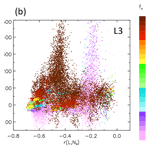# Quantifying albedo susceptibility biases in shallow clouds

Graham Feingold, Tom Goren, and Takanobu Yamaguchi
Abstract

The evaluation of radiative forcing associated with aerosol–cloud interactions remains a significant source of uncertainty in future climate projections. The problem is confounded by the fact that aerosol particles influence clouds locally and that averaging to larger spatial and/or temporal scales carries biases that depend on the heterogeneity and spatial correlation of the interacting fields and the nonlinearity of the responses. Mimicking commonly applied satellite data analyses for calculation of albedo susceptibility So, we quantify So aggregation biases using an ensemble of 127 large eddy simulations of marine stratocumulus. We explore the cloud field properties that control this spatial aggregation bias and quantify the bias for a large range of shallow stratocumulus cloud conditions manifesting a variety of morphologies and ranges of cloud fractions. We show that So spatial aggregation biases can be on the order of hundreds of percent, depending on the methodology. Key uncertainties emanate from the typically applied adiabatic drop concentration Nd retrieval, the correlation between aerosol and cloud fields, and the extent to which averaging reduces the variance in cloud albedo Ac and Nd. So biases are more often positive than negative and are highly correlated with biases in the liquid water path adjustment. Temporal aggregation biases are shown to offset spatial aggregation biases. Both spatial and temporal biases have significant implications for observationally based assessments of aerosol indirect effects and our inferences of underlying aerosol–cloud–radiation effects.

Share
Dates
1 Introduction

Shallow liquid clouds are a poorly quantified component of the climate system and one of the greatest sources of uncertainty for climate projections (e.g., Bony and Dufresne2005; Bony et al.2017). The problem is multifaceted and encompasses fundamental understanding of how these clouds are affected by the thermodynamic structure of the atmosphere, how they might change in a warmer world, how they are influenced by the atmospheric aerosol, and how all of these components are represented in climate models. The difficulty in quantifying the radiative effects of shallow clouds emanates, to a large extent, from the large range of spatiotemporal scales involved: aerosol–cloud interaction processes need to be understood and resolved at the scale of centimeters (e.g., Hoffmann et al.2019), while cloud fields and their organization are driven by larger-scale circulations at scales of hundreds to thousands of kilometers (e.g., Norris and Klein2000). Importantly, aerosol–cloud interactions acting at scales on the order of 100 m need to be resolved, (a) because they can lead to fundamental changes in the radiative state of a cloud system by changing the cloud albedo, cloud fraction, and spatial distribution of condensate and (b) because nonlinearities in aerosol–cloud–radiation interactions mean that the methodology of averaging small-scale properties to larger scales might generate biases in the radiative response.

This paper focuses on the ramifications of small-scale processes for cloud albedo susceptibility and cloud liquid water path adjustments (changes in liquid water path in response to changes in aerosol concentration) within the context of how they are treated in satellite-based analyses. Two key aspects are addressed: the first relates to spatial averaging from the level of the satellite pixel (order 1 km) to commonly used aggregation scales on the order of tens to hundreds of kilometers, and the second relates to temporal aggregation from the individual scene snapshot up to a timeframe on the order of months. Large-scale analyses often aggregate data both spatially and temporally into data sets that might cover, e.g., $\mathrm{4}{}^{\circ }×\mathrm{4}{}^{\circ }$ and 5 years , or a range of scales from kilometers to tens of kilometers for cloud microphysics, liquid water path, and radiation, $\mathrm{1}{}^{\circ }×\mathrm{1}{}^{\circ }$ for aerosol, and 3-month seasonal responses . While it is impractical not to aggregate to some degree, e.g., to smooth noisy retrievals or extract signals from a noisy background, the implications for quantifying aerosol–cloud interactions are still not well understood.

In the following, we will use the terms “aggregation” and “averaging” synonymously; “aggregation” tends to be used when speaking more broadly about including data from a larger range of spatial and temporal scales, whereas “averaging” is used in more of a mathematical sense.

We quantify aerosol–cloud interactions using the albedo susceptibility metric defined here as

$\begin{array}{}\text{(1)}& {S}_{\mathrm{o}}=\frac{d\mathrm{ln}{A}_{\mathrm{c}}}{d\mathrm{ln}{N}_{\mathrm{d}}}=\frac{\left(\mathrm{1}-{A}_{\mathrm{c}}\right)}{\mathrm{3}}\left[\mathrm{1}+\frac{\mathrm{5}}{\mathrm{2}}\frac{d\mathrm{ln}\mathcal{L}}{d\mathrm{ln}{N}_{\mathrm{d}}}\right],\end{array}$

where Ac is the cloud albedo, Nd is the drop concentration, and  is the liquid water path. The expression comprises the cloud brightening or Twomey component $\left(\mathrm{1}-{A}_{\mathrm{c}}\right)/\mathrm{3}$ and the adjustment term ${\mathcal{L}}_{\mathrm{o}}=d\mathrm{ln}\mathcal{L}/d\mathrm{ln}{N}_{\mathrm{d}}$ and assumes no changes in drop distribution width (e.g., Feingold and Siebert2009). The factor of $\mathrm{5}/\mathrm{2}$ suggests a potentially strong but uncertain contribution from  adjustments; even the sign of this term varies from positive for precipitating clouds to negative for non-precipitating clouds .

Using satellite-based observation systems, e.g., the MODerate Imaging Spectroradiometer (MODIS; Salomonson et al.1998), one can derive a drop concentration Nd,a, based on adiabatic assumptions, from retrieved visible cloud optical depth τ and cloud-top drop effective radius re:

$\begin{array}{}\text{(2)}& {N}_{\mathrm{d},\mathrm{a}}=\frac{\sqrt{\mathrm{5}}}{\mathrm{2}\mathit{\pi }k}{\left(\frac{{f}_{\mathrm{ad}}{c}_{\mathrm{w}}\left(T,P\right)\mathit{\tau }}{{Q}_{\mathrm{ext}}{\mathit{\rho }}_{\mathrm{w}}{r}_{\mathrm{e}}^{\mathrm{5}}}\right)}^{\mathrm{1}/\mathrm{2}},\end{array}$

where cw(T,P) is related to the condensation rate and is a known function of cloud-base temperature T and pressure P, fad is the adiabatic fraction (assumed in this paper to be 0.8), Qext is the extinction coefficient (≈2 in the visible part of the spectrum), ρw is the density of liquid water, and k is a factor that is inversely proportional to the width of the drop size distribution (assumed to be 0.8). When fad=1, Nd,a is the adiabatic drop concentration.

Liquid water path  is derived from MODIS data as

$\begin{array}{}\text{(3)}& \mathcal{L}=\frac{\mathrm{5}}{\mathrm{9}}{f}_{\mathrm{ad}}{r}_{\mathrm{e}}\mathit{\tau }.\end{array}$

Further details of these derivations can be found in and . Ac can be derived from τ using a simple two-stream approximation for a plane-parallel cloud (Bohren1987):

$\begin{array}{}\text{(4)}& {A}_{\mathrm{c}}=\frac{\mathit{\tau }}{\mathit{\gamma }+\mathit{\tau }},\end{array}$

where γ depends on the degree of forward scattering. Equation (4) also assumes an overhead sun, no absorption, and a dark underlying surface. We do not consider three-dimensional radiative transfer.

As an example of how averaging of data can affect the quantification of derived variables, we note that Eq. (4) is a concave function, which, following Jensen's inequality, means that for an inhomogeneous cloud field, $f\left(\stackrel{\mathrm{‾}}{\mathit{\tau }}\right)>\stackrel{\mathrm{‾}}{f\left(\mathit{\tau }\right)}$. Thus, calculating τ based on large length-scale-averaged cloud properties and then calculating ${A}_{\mathrm{c}}=f\left(\stackrel{\mathrm{‾}}{\mathit{\tau }}\right)$ using Eq. (4) will generate a high bias in Ac that is inherently a function of the inhomogeneity of the cloud field. Because this well-known albedo bias is not the topic of this paper, we will assume in all calculations that Ac is measured directly by an instrument like Clouds and the Earth's Radiant Energy System (CERES) at the desired measurement length scale and therefore does not suffer from an averaging bias. Instead, we explore similar biases that affect quantification of So. For example, Eq. (2) is a highly nonlinear function of τ and re so that whether one elects to calculate Nd,a before or after averaging of component variables τ and re will potentially have a strong effect on So.

analyzed large eddy simulation (LES) output of three cloud fields characterized by different degrees of inhomogeneity and showed that an aerosol–cloud interaction metric, $d\mathrm{ln}\mathit{\tau }/d\mathrm{ln}{N}_{\mathrm{d}}$, increased as a result of averaging – more so for heterogeneous fields than for homogeneous fields. To put the topic on firmer footing, we first establish a theoretical framework for assessing the biases. We then use a large number of LESs (127) as proxy data, which we use to simulate satellite retrievals. We apply typical methodologies used in satellite retrievals, as well as variants, to assess the provenance of the So bias. Both spatial and temporal aggregation scales are considered, with a focus on the former. Because of the limited domain size available to LES, we concern ourselves with the effects of spatial averaging from scales on the order of 1 to 10 km. The multiple snapshots of different scenarios available from the LES output provide the basis for temporal aggregation. We note that also looked at the effect of aggregation scale on quantification of aerosol indirect effects (the radiative forcing of aerosol–cloud interactions). Their analysis addressed much larger scales (1, 4, and 60) in climate models. Here we focus on a model framework that explicitly resolves aerosol–cloud interactions at the cloud scale.

2 Theory

## 2.1 Spatial aggregation of variables derived from nonlinear functions

The two fundamental geophysical variables associated with aerosol–cloud interactions are N (a generic concentration or drop or aerosol number concentration, which are well correlated) and . In the case of homogeneous aerosol and cloud fields, averaging of data to different scales has no effect on derived quantities such as Nd, , Ac, and So, and the order of calculation of these fields is of no consequence. In reality, however, cloud fields exhibit different degrees of inhomogeneity: condensation of cloud water responds to local updrafts and, to some extent, availability of cloud condensation nuclei. Drop concentration depends on aerosol concentration – typically a less variable field than cloud water – as well as local supersaturation driven by updrafts. Under these conditions, the quantification of aerosol–cloud interaction metrics like So and the influence of averaging could be far more important.

The theoretical framework for addressing this question is well known from similar examples in the atmospheric sciences, notably biases in rain formation processes that result from large-scale averaging of the cloud water and drop concentration terms in expressions for autoconversion and accretion (e.g., Lebsock et al.2013; Zhang et al.2019). In the interest of completeness, we repeat the key equations here. Assuming a lognormal probability distribution function (PDF) of quantity x,

$\begin{array}{}\text{(5)}& P\left(x\right)=\frac{\mathrm{1}}{\sqrt{\mathrm{2}\mathit{\pi }}x\mathrm{ln}{\mathit{\sigma }}_{\mathrm{g},x}}\mathrm{exp}\left[-\left(x-{x}_{\mathrm{g}}\right)/\left(\mathrm{2}{\mathrm{ln}}^{\mathrm{2}}{\mathit{\sigma }}_{g,x}\right)\right],\end{array}$

where the lognormal parameters are geometric mean (or median) xg and geometric standard deviation σg,x. Quantity x represents Ac or  and N. Using well-known integral properties of the lognormal function (e.g., Feingold and Levin1986), it is easy to show that the bias in a moment ${x}^{{\mathit{\beta }}_{x}}$ associated with averaging can be written as

$\begin{array}{}\text{(6)}& {B}_{x}=\frac{\stackrel{\mathrm{‾}}{{x}^{{\mathit{\beta }}_{x}}}}{{\stackrel{\mathrm{‾}}{x}}^{{\mathit{\beta }}_{x}}}={\left({D}_{x}^{\mathrm{2}}+\mathrm{1}\right)}^{\frac{{\mathit{\beta }}_{x}^{\mathrm{2}}-{\mathit{\beta }}_{x}}{\mathrm{2}}},\end{array}$

with the relative dispersion Dx, the ratio of the standard deviation to the mean, defined as

$\begin{array}{}\text{(7)}& {D}_{x}={\left(\stackrel{\mathrm{‾}}{\left(x-\stackrel{\mathrm{‾}}{x}{\right)}^{\mathrm{2}}}\right)}^{\mathrm{1}/\mathrm{2}}/\stackrel{\mathrm{‾}}{x}.\end{array}$

If the two interacting fields, e.g.,  and N, are assumed to follow a bivariate lognormal distribution, the bias associated with the covariance between  and N is

$\begin{array}{}\text{(8)}& {B}_{\mathrm{cov}}=\mathrm{exp}\left(r\left(\mathcal{L},N\right)\cdot {\mathit{\beta }}_{\mathcal{L}}\cdot {\mathit{\beta }}_{N}\cdot {\mathit{\sigma }}_{\mathrm{g},\mathcal{L}}\cdot {\mathit{\sigma }}_{\mathrm{g},N}\right),\end{array}$

where r(ℒ,N) is the spatial correlation between  and N. (Note that we elect to present the theory in terms of  and N rather than Ac and N because Ac includes compounded dependence on N via τ. Since  and Ac are highly correlated, this choice does not affect the general framework for discussion.)

The overall bias associated with averaging for two covarying fields is then given by

$\begin{array}{}\text{(9)}& B={B}_{\mathcal{L}}\cdot {B}_{N}\cdot {B}_{\mathrm{cov}}.\end{array}$

The equations allow theoretical calculation of the So biases associated with two covarying fields  and N, each characterized by its own heterogeneity D and DN, respectively.

To apply this analysis to biases in So associated with interacting  and N fields, we make a number of assumptions. In the absence of an analytical form for the  adjustment, we assume it is negligible. We also assume that Ac depends linearly on τ, which holds over a large range of τ. So is then approximated as being proportional to 1−τ (Eq. 1). Note that because the percent bias in 1−τ is equivalent to the percent bias in τ, we can apply

$\begin{array}{}\text{(10)}& \mathit{\tau }=c{\mathcal{L}}^{\mathrm{5}/\mathrm{6}}{N}^{\mathrm{1}/\mathrm{3}}\end{array}$

(e.g., Brenguier et al.2000), where c is a constant that does not require specification for the purpose of the current analysis. Then, with ${\mathit{\beta }}_{\mathcal{L}}=\mathrm{5}/\mathrm{6}$ and ${\mathit{\beta }}_{N}=\mathrm{1}/\mathrm{3}$, Eq. (9) can be calculated for given values of D, DN, and r(ℒ, N). These are shown in Fig. 1 in D; DN space for three values of r(ℒ, N). We note that large positive and negative biases can result from averaging; for negative r(ℒ, N), biases are positive and on the order of 20 %–60 %, whereas for positive values of r(ℒ,N), biases are negative and on the order of −20 % to −70 %. When correlation between the fields is zero, biases are 10 %–20 %. The central role of r(ℒ, N) is further illuminated by plotting the bias in So as a function of r(ℒ, N) for specified D and DN combinations (Fig. 2).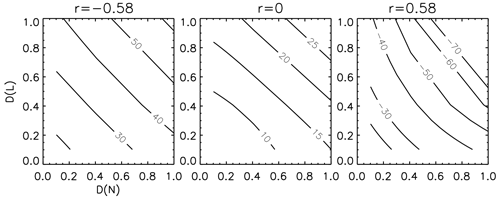Figure 1Theoretical calculations of the albedo susceptibility bias (%) for a range of relative dispersions in  and N (D(ℒ) and D(N), respectively) for three different correlations (r) between  and N. Note the values of large positive and negative biases.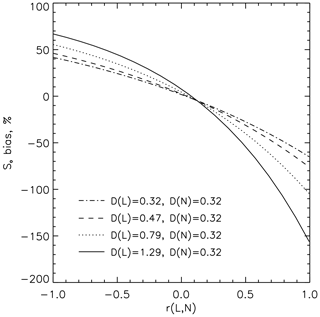Figure 2As in Fig. 4 but for the So bias as a function of the spatial correlation between  and N for a range of relative dispersions in  (D(ℒ)) and fixed D(N). Of note is that for the conditions shown the biases are large and range from about −150 % (positive r, large D(ℒ)) to +70 % (negative r, large D(ℒ)).

Note that the assumption of a bivariate lognormal distribution is common when dealing with geophysical fields. Another cautionary note is that, given the various assumptions applied to generate the results in Figs. 1 and 2, they should be considered illustrative; i.e., they are primarily intended to highlight key variables that control aggregation bias. When we embark on our analysis of LES output, we will assume that τ and re are known exactly, and quantitative comparison with Figs. 1 and 2 should be avoided.

## 2.2 Effects of spatial and temporal averaging on variance, and correlation between fields

The second framework for assessment of biases derives from the basic definition of the linear regression fit:

$\begin{array}{}\text{(11)}& \stackrel{\mathrm{^}}{b}={r}_{x,y}\frac{{\mathit{\sigma }}_{y}}{{\mathit{\sigma }}_{x}},\end{array}$

where $\stackrel{\mathrm{^}}{b}$ is the regression slope and σx is the standard deviation of field x. In our case, x is aerosol or drop concentration (we will use Nd) and y is the cloud variable (Ac or ). As shown by , averaging increases r(x,y) but decreases σx and σy to varying degrees. Of interest is therefore the extent to which averaging changes r(x,y) and the ratio ${\mathit{\sigma }}_{y}/{\mathit{\sigma }}_{x}$.

3 Large eddy simulation as a data source

We calculate So biases using output from 127 large eddy simulations of marine stratocumulus under a range of conditions from fairly homogeneous overcast to broken open-cellular structures.

Simulations are generated by the System for Atmospheric Modeling . Input conditions are derived from ERA-5 reanalysis in the stratocumulus regime off the coast of California. The model setup is similar to and , with initial meteorological and aerosol conditions sampled using the Latin hypercube method. Simulations are nocturnal and of 12 h duration. The first 5 h of output from each simulation is discarded to avoid cloud scenes that are not fully developed. The domain size is 48 km × 48 km × 2.5 km with a 500 m damping layer below the model top at 2.5 km. The profile is extended up to 35 km (5 hPa) for radiation calculations. Grid spacings are $\mathrm{d}x=\mathrm{d}y=\mathrm{200}$ m and dz=10 m. A two-moment bulk microphysical method that calculates all warm cloud processes (including supersaturation and activation) is applied . The simulations differ from in one key respect, namely, that surface fluxes are calculated interactively. Resultant cloud fields exhibit varying degrees of heterogeneity, including closed-, open-, and transitions from closed- to open-cellular convection.

The LES output provides microphysical fields of drop number concentration and liquid water content as three-dimensional prognostic variables under the assumption of a bimodal lognormal distribution of cloud drops and raindrops with fixed distribution width (σg=1.2 for both). However, to mimic satellite retrievals, we work with the derived model variables τ and cloud-top re to calculate Nd and  based on Eqs. (2) and (3). Both cloud water and rainwater contribute to τ and re. Cloud top is calculated based on a liquid water mixing ratio threshold (0.01 g kg−1). Because these clouds are strongly capped, the first grid point exceeding this value (when working from above and downward towards the cloud top) almost always exceeds the threshold significantly, providing a maximum or near-maximum re in each model column. Ac is calculated based on the modeled value of τ (Eq. 4), is then averaged, and therefore does not suffer from averaging bias. So is calculated directly for each scene based on the definition (${S}_{\mathrm{o}}=d\mathrm{ln}{A}_{\mathrm{c}}/d\mathrm{ln}{N}_{\mathrm{d}}$) using least squares regression to the natural logarithms of Ac and Nd.

## 3.1 Spatial aggregation

### 3.1.1 Level-2 analysis

The standard averaging method follows the MODIS level-2 (L2) methodology in the sense that calculations are performed at high resolution prior to averaging. In the current work, Eqs. (2) and (3) are calculated based on the native 200 m model mesh (n=1) and then averaged to n×n tiles. Results will be shown for n=30 vs. n=4, i.e., 6 km × 6 km boxes vs. 800 m × 800 m boxes. These choices result from a desire for a reasonable number of regression points (n=30) and for some small amount of smoothing (n=4) to reduce the noise in Nd retrievals (Eq. 2). The choice of n=4 also brings us close to the typical 1 km length scale used for analysis of L2 MODIS data. (We did not use n=5, i.e., 1 km, because the number of points in the domain is even, and our desire is to maximize our coverage and simplify analysis.) Note that L2 methodology removes the biases associated with nonlinear functions applied to averaged data discussed in Sect. 2.1 (Jensen's inequality) since Nd is calculated using Eq. (2) at the 200 m level and then averaged up. The same is true for Ac, which as previously noted is calculated based on Eq. (4).

Biases are defined as

$\begin{array}{}\text{(12)}& \left(\stackrel{\mathrm{‾}}{X}-X\right)/X,\end{array}$

where X represents So calculated at n=4 and $\stackrel{\mathrm{‾}}{X}$ represents So at n=30. Correlations r(ℒ,Nd) refer to calculations for n=4 unless otherwise stated. Nd in the L2 analysis represents cloudy-column averaged drop concentration, i.e., ${N}_{\mathrm{d}}={N}_{\mathrm{d},\mathrm{a}}$ based on Eq. (2).

The Nd retrieval (Eq. 2) is very sensitive to thin clouds, particularly when re but also τ are small. As is common in MODIS data analyses, calculations are only applied to thicker clouds, in our case to re>3µm and τ>3.

### 3.1.2 Variants

Two variants of the calculations will be presented.

1. Mimic MODIS level-3 (L3) analysis. Here satellite-based retrievals are based on aggregated data. In other words, Eqs. (2) and (3) for Nd and , respectively, are applied to data averaged to n=4 and n=30. By doing so the averaging biases associated with Jensen's inequality are introduced. The biases are expected to derive from a mix of influences: low for Nd (a convex function in re dominates a concave function in τ; Eq. 2) and negligible for  (Eq. 3). The effect of smoothing associated with level-3 aggregation is thus likely to be highly dependent on cloud field heterogeneity.

2. Mimic MODIS L2 analysis but eliminate uncertainties in the (sub)adiabatic retrieval of Nd by assuming a “perfect” Nd, which is taken directly from the LES. Because the LES Nd is a three-dimensional variable, we use in-cloud, column average values. This methodology will be referred to as L2N. Note that  is still calculated as per Eq. (3). The goal here is to understand the extent to which the retrieval of Nd drives the regression biases.

## 3.2 Temporal aggregation

To address temporal aggregation, we consider the same individual LES snapshots described above but calculate So in two ways: (i) So regression fits to individual cloud scenes are simply averaged up over all cloud scenes, and (ii) LES output is temporally aggregated to a large data set from which So is calculated via regression. The first approach preserves the individual cloud scene susceptibilities, while the second aggregates many different cloud fields before performing the regression. The biases are calculated based on Eq. (12), with the overbar indicating the second approach (ii). The methodology is followed (separately) for both n=4 and n=30 spatial averaging and for L2, L3, and L2N.

4 Results

## 4.1 Spatial aggregation

### 4.1.1 Effect of spatial aggregation on So and correlation

Figure 3 presents results for the three approaches (L2, L3, and L2N). We start with the figures showing ${\stackrel{\mathrm{‾}}{S}}_{\mathrm{o}}$ vs. So to avoid ambiguity in the sign of the bias associated with Eq. (12). (According to Eq. 12, conditions under which ${\stackrel{\mathrm{‾}}{S}}_{\mathrm{o}}$ is more negative than So also manifest as positive biases.) The solid line is the 1:1 line.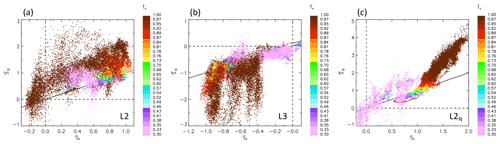Figure 3So aggregated to a 6 km scale (${\stackrel{\mathrm{‾}}{S}}_{\mathrm{o}}$) vs. So aggregated to an 800 m scale (So) for the (a) L2, (b) L3, and (c) L2N methodologies as described in the text. Solid line is the 1:1 line. Dashed lines are drawn at ordinate and abscissa values of zero. Note the general overestimate in So with increasing aggregation scale in (a) and (c) and the change in sign in So associated with L3 aggregation in (b). Of note is that high-cloud-fraction/low-heterogeneity conditions are often associated with high biases.

Points are colored by cloud fraction fc (defined at the native 200 m grid spacing) since it also serves as a good proxy for cloud field heterogeneity. (The correlation between fc and D(ℒ) is 0.86.) For L2, we note that both ${\stackrel{\mathrm{‾}}{S}}_{\mathrm{o}}$ and So are almost always positive and that low-fc states do not suffer from worse biases than high-fc states. By contrast, high fc is often associated with the largest biases. The reasons for this will become apparent in the subsequent analysis.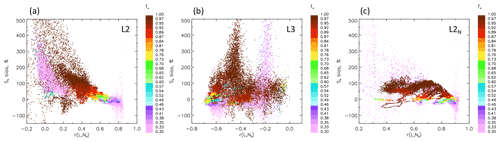Figure 4So bias in percent as defined in Eq. (12) as a function of the correlation between liquid water path and drop concentration r(ℒ, Nd) with points colored by cloud fraction fc for the (a) L2, (b) L3, and (c) L2N methodologies. In (a) and (c) the So bias tends to increase with decreasing r(ℒ, Nd) and increasing cloud fraction fc, with noted exceptions. Use of the true Nd in (c) restricts the So bias to about 100 %.

Responses for the L3 analysis are distinctly different in a number of ways: first, both ${\stackrel{\mathrm{‾}}{S}}_{\mathrm{o}}$ and So are almost always negative, and low-fc cloud scenes often have lower biases than high-fc scenes. This is because L3 averaging has a stronger smoothing effect on broken cloud fields and therefore somewhat unexpectedly reduces the averaging bias for broken cloud fields compared to solid cloud fields. Nevertheless, the non-physical shift in the sign of So associated with the L3 methodology should act as a cautionary note. We present an explanation for the reversal of sign in Appendix A.

L2N analysis yields strongly positive So and a clearer dependence of the bias on fc. For broken cloud scenes So is sometimes negative, but biases tend to be scattered and relatively small. These low fc≈0.3 states are dominated by cumulus cells with stronger updrafts that result in coherent Nd. Since So and ${\stackrel{\mathrm{‾}}{S}}_{\mathrm{o}}$ are only calculated in cloudy regions (above the re and τ thresholds), this coherence in Nd results in a small bias. Thus the reasons for small susceptibility bias at low fc differ for L3 and L2N. With increasing fc, biases tighten around the 1:1 line but start to deviate for fc>0.85 and exhibit increasingly large values.

To quantify the biases, these same analyses are shown as percent biases in So (Eq. 12) as a function of the correlation between  and Nd (r(ℒ, Nd); Fig. 4), the calculations of which are consistent with the derivation of variables averaged to n=4; e.g., L2 and L3 calculations apply r(ℒ, Nd) based on Eqs. (2) and (3), and L2N calculates r(ℒ, Nd) using the true Nd and Eq. (3).

L2 biases are almost always positive and can reach values of many hundreds of percent (Fig. 4). As expected from Sect. 2.1, r(ℒ, Nd) has a strong influence on the So bias, particularly for L2, with the bias increasing noticeably with decreasing r(ℒ, Nd) in a manner qualitatively similar to Fig. 2. The high values and high variability in the bias as one approaches r(ℒ, Nd)≈0 are to some extent a consequence of an uncertain regression fit when the correlation between the  (or the closely related Ac) and Nd fields is poor.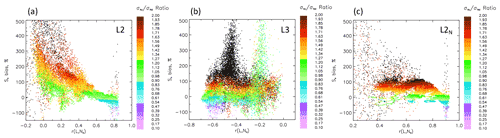Figure 5As in Fig. 4 but with points colored by the ratio of ${\mathit{\sigma }}_{{A}_{\mathrm{c}}}/{\mathit{\sigma }}_{{N}_{\mathrm{d}}}$ at 6 km to ${\mathit{\sigma }}_{{A}_{\mathrm{c}}}/{\mathit{\sigma }}_{{N}_{\mathrm{d}}}$ at 800 m (the σ ratio). Note the clear dependence of the So bias on the σ ratio and that the bias ∼0 when the σ ratio ∼1 (green colors). Exceptions to the latter occur for L2 at low r(ℒ, Nd).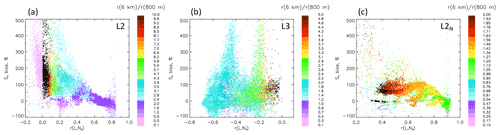Figure 6As in Fig. 4 but with points colored by the ratio of correlations r(ℒ, Nd) at 6 km to r(ℒ, Nd) at 800 m (the r ratio). Comparison with Fig. 5 shows approximately orthogonal dependence of the σ and r ratios on the So bias. In (a) and (b), calculation of the r ratio is mathematically unstable at r(ℒ, Nd)=0, as evidenced by saturating colors.

Of note is that the L3 analysis methodology (aggregate first and then derive) changes the sign of r(ℒ, Nd) to negative values, as it did the sign of So (Fig. 3). L3 biases are more widely dispersed and show no clear trend with r(ℒ, Nd) or fc. L2N biases tend to be capped at about 100 %, and values of r(ℒ, Nd) are noticeably more positive than those in L2. These results reinforce two points, (i) that L3 analysis generates non-physical results, negative So, and a change in the sign of r(ℒ, Nd), and (ii) that the use of the (sub)adiabatic assumption (Eq. 2) as a proxy for Nd incurs a significant increase in the So bias relative to L2N, most noticeably at low r(ℒ, Nd), where Eq. (2) results in a significantly reduced (but for the most part positive) correlation.

### 4.1.2 Effect of spatial aggregation on regression

It is useful to turn to the underlying definitions of regression analysis (Sect. 2.2) to explore more deeply the influence of averaging on the So bias. According to Eq. (11), So biases are related to the ratio of $\stackrel{\mathrm{^}}{b}$ (6 km) to $\stackrel{\mathrm{^}}{b}$ (800 m):

$\begin{array}{}\text{(13)}& \stackrel{\mathrm{‾}}{\stackrel{\mathrm{^}}{b}}/\stackrel{\mathrm{^}}{b}=\frac{{\stackrel{\mathrm{‾}}{r}}_{x,y}}{{r}_{x,y}}\left(\frac{{\stackrel{\mathrm{‾}}{\mathit{\sigma }}}_{y}}{{\stackrel{\mathrm{‾}}{\mathit{\sigma }}}_{x}}\right)/\left(\frac{{\mathit{\sigma }}_{y}}{{\mathit{\sigma }}_{x}}\right),\end{array}$

with $\stackrel{\mathrm{‾}}{\stackrel{\mathrm{^}}{b}}$ denoting 6 km averaging and $\stackrel{\mathrm{^}}{b}$ denoting 800 m averaging. We therefore consider (i) the ratio ${\stackrel{\mathrm{‾}}{\mathit{\sigma }}}_{{A}_{\mathrm{c}}}/{\stackrel{\mathrm{‾}}{\mathit{\sigma }}}_{{N}_{\mathrm{d}}}$ to ${\mathit{\sigma }}_{{A}_{\mathrm{c}}}/{\mathit{\sigma }}_{{N}_{\mathrm{d}}}$ (henceforth the “σ ratio”; Fig. 5) and (ii) the ratio $\stackrel{\mathrm{‾}}{r}\left({A}_{\mathrm{c}},{N}_{\mathrm{d}}\right)/r\left({A}_{\mathrm{c}},{N}_{\mathrm{d}}\right)$ (henceforth the “r ratio”; Fig. 6), which by Eq. (11) are both determinants of the bias. Note that, for the r ratio, we use r(Ac, Nd) to adhere more rigorously to the regression analysis definitions. The interpretation of these ratios is non-trivial. They express the extent to which ${\mathit{\sigma }}_{{A}_{\mathrm{c}}}/{\mathit{\sigma }}_{{N}_{\mathrm{d}}}$ and r(Ac, Nd) are modified by averaging. In the case of ${\mathit{\sigma }}_{{A}_{\mathrm{c}}}/{\mathit{\sigma }}_{{N}_{\mathrm{d}}}$ this amounts to interpreting a “ratio of ratios”. Later we will delve into this in more detail.

L2 results show a clear dependence of the So bias on the σ ratio and especially large biases when the σ ratio is high (Fig. 5a). These also happen to be points exhibiting high fc (cf. Fig. 4a). Also apparent is that the separation of positive and negative biases is demarcated at a σ ratio of 1. The results suggest a strong correlation between the σ ratio and fc. At high r(ℒ, Nd), the So bias increases systematically with increasing σ ratio (Fig. 5a), but with decreasing r(ℒ, Nd) the strong and orthogonal influence of the r ratio becomes more important (Fig. 6a). Clearly evident in Fig. 6a is the anticipated unstable calculation of the r ratio in the vicinity of r(ℒ, Nd)=0.

The L3 methodology exhibits an even clearer dependence of the So bias on the σ ratio, except for cloud fields with r(ℒ, ${N}_{\mathrm{d}}\right)\approx -\mathrm{0.2}$ (Fig. 5b), where the high bias is clearly related to both the σ and r ratios (Fig. 6b). The aforementioned vertically oriented green-colored points have a σ ratio of about 1 and an r ratio of 2.5; i.e., the bias is driven by the r ratio.

For the L2N methodology, here too the σ ratio (Fig. 5c) provides a clearer indication of the magnitude of the bias compared to either the r ratio (Fig. 6c) or fc (Fig. 4).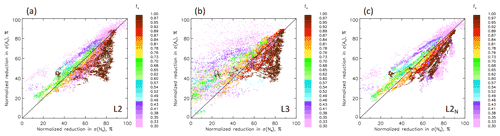Figure 7Effects of aggregation on normalized reduction in σ(Ac) vs. normalized reduction in σ(Nd) to shed light on results in Fig. 5. Points are colored by fc. (a–c) At high (low) fc averaging tends to more significantly reduce (increase) σ(Nd) relative to σ(Ac). Notable exceptions occur in (c) for some low-fc conditions. See text for further discussion.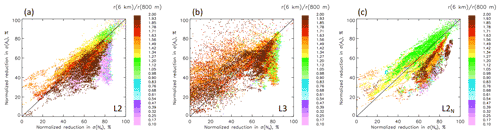Figure 8As in Fig. 7 but with points colored by the r ratio. See text for discussion.

Based on Eq. (13), the influence of the σ and r ratios on So bias is expected. What is more revealing is the influence of averaging on the components of these ratios. To this end, Fig. 7 examines the effect of averaging on the reduction in ${\mathit{\sigma }}_{{A}_{\mathrm{c}}}$ and ${\mathit{\sigma }}_{{N}_{\mathrm{d}}}$. In other words, we ask to what extent averaging smoothens the Ac field relative to the smoothing in the Nd field. Accordingly, axes in Fig. 7 are calculated as normalized reductions in the variables.

L2 analysis shows that at low fc the normalized reductions in σ(Nd) tend to be smaller than those in σ(Ac) but that the reverse tends to be true for fc>0.75 (Fig. 7a); i.e., at high fc, averaging smoothens the Nd field more than it smoothens the Ac field. We will show below that these high-fc scenes, although inherently more homogeneous, often manifest significant inhomogeneity in Nd as a result of the Nd retrieval. (See further discussion in Sect. 4.1.4, Examples.)

The clear exception to the trend of averaging and smoothing Nd more than Ac with increasing fc is the group of low-fc points that exists in Fig. 4a at $r\left(\mathcal{L},{N}_{\mathrm{d}}\right)<\mathrm{0.2}$. These anomalous points appear below the 1:1 line in Fig. 7a and show up as lower r-ratio points (reddish points) in a sea of higher r-ratio points (brown colors) in Fig. 8a. Another distinct feature is the group of vertically oriented high-fc (Fig. 7a) and low r-ratio (Fig. 8a) points for which smoothing of Nd increasingly exceeds smoothing in Ac as one moves below the 1:1 line. Because these points are characterized by small values of the r ratio, there appears to be an offsetting of σ-ratio and r-ratio effects.

A closer look shows that these points manifest as a negative So bias (Fig. 9a); in other words, the reduction in the r ratio dominates the increase in the σ ratio. Although these negative bias points tend to be more rare, they can be identified in Fig. 4a at high fc and low r(ℒ, Nd) (<0.3).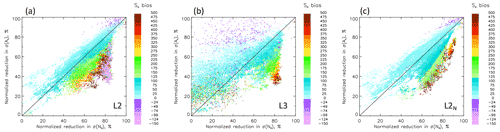Figure 9As in Fig. 7 but with points colored by So bias. Biases exceeding 100 % always occur when averaging-related smoothing has a stronger effect on Nd than on Ac.

Analysis of L3 shows some similarities to and some differences from L2. First, there is a much more significant scatter in points, particularly at lower fc (Fig. 7b); second, as in L2, averaging-related smoothing of Nd tends to exceed smoothing in Ac at higher fc (Fig. 7b); third, and different from L2, values of the r ratio of ≈1 (Fig. 8b, green colors) are associated with higher So biases (Fig. 9b), which must derive from the σ ratio.

Finally, L2N reveals a somewhat richer palette of responses. First the commonalities: (i) the general trend for smoothing of Nd to exceed smoothing of Ac with increasing fc and increasing r ratio is relatively robust (cf. Fig. 7a–c). (ii) When the r ratio  1 and smoothing of the fields is similar (Fig. 8a and c), the So bias is capped at about 100 % (Fig. 9a and c). In fact it is clear from Fig. 9 that So biases exceeding 100 % always occur when averaging-related smoothing has a stronger effect on Nd than on Ac, although the magnitude and even sign of these biases vary significantly depending on the methodology. The richness (lack of monotonicity) in responses under these conditions depends on the relative strength of the r ratio and the extent to which it amplifies or counteracts the σ ratio.

We note one interesting difference between L2 and L2N: for the latter, low-fc points reside in conditions under which averaging-related smoothing is dominated by more as well as less significant smoothing of Nd vs. Ac (Fig. 7c). In very rare cases the low-fc points above but close to the 1:1 line in Fig. 9c cause negative So biases (cf. Fig. 6c). Finally, some very high positive So biases (up to 500 %) do exist for L2N. These can be traced to conditions when the r ratio is large (Fig. 8c), and averaging smoothens Nd more than Ac. In this case the effects of averaging on the r ratio and the σ ratio work in unison to amplify the bias.

### 4.1.3So bias vs. ℒ adjustment bias

The topic of  adjustments is of great interest given that the term may both enhance or offset the overall albedo susceptibility (Eq. 1) (e.g., Glassmeier et al.2021). For example, a value of ${\mathcal{L}}_{\mathrm{o}}=d\mathrm{ln}\mathcal{L}/d\mathrm{ln}{N}_{\mathrm{d}}<-\mathrm{0.4}$ will change the sign of So from positive to negative. Numerous recent articles, based on models and observations, point to o being positive in precipitating conditions, following the familiar “cloud lifetime” hypothesis which posits that aerosol perturbations will suppress collision–coalescence and decrease precipitation and therefore  losses, while it is negative in the non-precipitating regime as a result of enhanced evaporation–entrainment feedbacks . Figure 10 shows the relationship between spatial-averaging-related So and o biases, with points colored by fc. For clarity we show a subsample of 58 of the total 127 simulations to avoid points clustering and obscuring points below. These 58 samples represent Latin hypercube sampling of the full data set and do not exhibit any bias relative to the full set.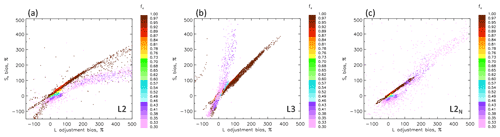Figure 10So bias vs. the  adjustment bias ($d\mathrm{ln}\mathcal{L}/d\mathrm{ln}{N}_{\mathrm{d}}$ in Eq. 1) for a limited set of model scenes (for clarity). Note a strong positive correlation between the two biases, as expected from Eq. (1). In (a) and (b), the relative magnitude of these biases depends on fc, although with an opposite trend. In (a) and (c), the  adjustment bias tends to be larger than the So bias.

First, we note a strong positive correlation between the two biases, with o biases larger than So biases in L2 and L2N (Fig. 10a and c). The reverse is true for L3 (Fig. 10b). For L2 and L3, two distinct branches appear: the first is a close relationship for fc>0.7, while the second is somewhat less well defined and associated with lower fc. There is a saturation in the ratio for fc on the order of 0.3 in L2 (Fig. 10a), while L3 shows a distinctly stronger So bias at low fc compared to the approximately linear relationship for high fc (Fig. 10b). Detailed analysis of this flip in the relative slope for high fc vs. low fc for L2 and L3 can be traced to relative differences in the degree of aggregation-related smoothing between Ac and  (see Appendix B).

The separation of these branches is much less distinct for L2N (Fig. 10c), but in general o biases are larger than So biases, although to a lesser extent than in L2. The absence of a clear separation in the branches is a result of the use of the correct Nd such that cloud heterogeneities affect the biases to a similar degree. While the strong positive correlation between So and o biases is not surprising given the expected close relationship between So and o, it is clear that, using the methodologies applied here, satellite-based analyses of the o bias have the potential to be at least as severe as those associated with So biases.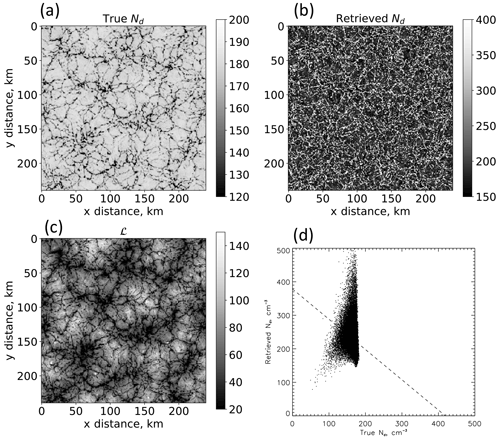Figure 11High-resolution (n=1) two-dimensional snapshot of (a) “true” Nd, (b) retrieved Nd (Eq. 2), (c) , and (d) the relationship between (b) and (a). Note the different scales between (a) and (b). Although the mean values of Nd in (a) and (b) differ by only −36 %, they exhibit negative correlation over the scene. The retrieved Nd introduces significant heterogeneity into the field. Use of the true Nd reduces the absolute value of the So error from −2017 % to +80 %.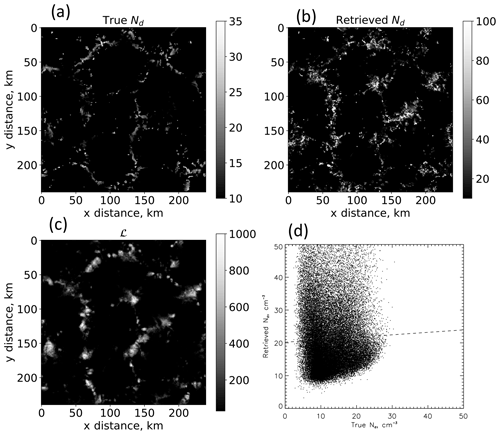Figure 12As in Fig. 11 but for an open-cellular case. Note the different scales between (a) and (b). Mean values of Nd in (a) and (b) differ by −75 %. Use of the true Nd degrades the So bias (285 % for L2 vs. 803 % for L2N).

### 4.1.4 Examples

While our goal has been to provide a broad assessment of susceptibility biases in terms of cloud field properties, some examples are helpful for illustrating the issues. We focus on L2 and L2N to isolate the effect of the Nd retrieval for individual cases, chosen randomly based on their visual physical characteristics but supported by other cases. Figure 11 presents an LES-generated stratocumulus scene characterized by high fc (=0.98) and a very realistic closed-cellular structure (Fig. 11a and c). Drop concentration fields for the (sub)adiabatically derived Nd, i.e., Nd,a (Eq. 2) and the “true” (LES) Nd show the problem very clearly. The retrieved Nd shows a great deal more fine-scale structure than the true Nd, and although in the mean the retrieved Nd is not highly unrealistic (retrieved Nd=167 cm−3; true Nd=227 cm−3 – an error of −26 %), the two fields are strongly negatively correlated, with a fit slope of Nd (retrieved) =$\mathrm{378}-\mathrm{0.9}×{N}_{\mathrm{d}}$ (true). To quantify this further, we consider the correlations between  and Nd, applying the true Nd, r(ℒ, Nd)=0.78 while using the retrieved Nd, r(ℒ, ${N}_{\mathrm{d}}\right)=-\mathrm{0.24}$. This shift from strong positive r(ℒ, Nd) to negative r(ℒ, Nd) has implications for the So bias (e.g., Fig. 2): the L2-derived So bias is −2017 %, whereas the L2N bias is +80 %. Here the use of the true Nd reduces the So error very significantly. This example serves to explain why high So biases can exist in high-fc scenes (e.g., Fig. 4). We emphasize that the significant inhomogeneity in Nd is a result of the Nd retrieval rather than an inherent property of the cloud field and that it is this increase in inhomogeneity and reduction in r(ℒ, Nd) that drives up the So bias.

The second example (Fig. 12) is a low-fc case (0.23) exhibiting classic open-cellular structure. Here the mean retrieved Nd is 21 cm−3 and the true Nd is 12 cm−3 – an error of +75 %. The best-fit linear regression between the two yields Nd (retrieved) =$\mathrm{20}+\mathrm{0.075}×{N}_{\mathrm{d}}$ (true). Examining the true Nd, we find r(ℒ, Nd)=0.13, whereas, when using the retrieved Nd, r(ℒ, ${N}_{\mathrm{d}}\right)=-\mathrm{0.05}$. The So biases are 285 % and 803 % for L2 and L2N, respectively; i.e., the true Nd degrades the So bias. We have identified two contributing factors to this unexpected result: (i) in the case of L2N, the proximity of r(ℒ, Nd) to zero generates an unstable r ratio and an unstable So bias (Eq. 13); (ii) more generally, unexpected results can occur when the base So (n=4) is small, which by Eq. (12) will generate unstable bias calculations. For the current case, So is small because the open cell walls are already very bright; i.e., 1−Ac in Eq. (1) is small.

Note that the results in Fig. 12 may seem counterintuitive at first glance since, from a satellite remote sensing perspective, a negative bias in retrieved Nd is generally expected in broken cloud scenes due to a positive bias in re and a negative bias in τ (e.g., Grosvenor et al.2018). However, the aforementioned bias is a satellite-based measurement bias. In our case, we assume that re and τ are correct (taken directly from the model). We take the opportunity to emphasize that this work focuses on the effect of averaging cloud fields characterized by different morphologies and not with the broken cloud re and τ biases. The latter would need to be considered with a MODIS instrument simulator for a more complete assessment.

While examination of case studies proves useful, we argue that the broader statistical view is essential to understanding the error landscape that might be encountered in highly variable natural cloud scenes. In this regard, Figs. 36, supported by Figs. 79, are essential.

Table 1Temporal averaging values and percent differences. ΣSo refers to a simple average of the So for individual cloudy scenes. ${\stackrel{\mathrm{‾}}{S}}_{\mathrm{o}}$ refers to the So regression fit to the temporally aggregated data from all cloudy scenes. The percent difference is defined as (${\stackrel{\mathrm{‾}}{S}}_{\mathrm{o}}-\mathrm{\Sigma }{S}_{\mathrm{o}}\right)/\mathrm{\Sigma }{S}_{\mathrm{o}}$. n=4 and n=30 refer to the 800 m and 6 km spatial averaging scales, respectively.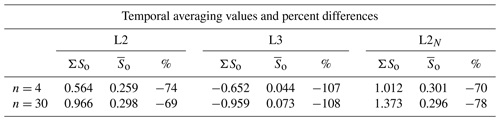## 4.2 Temporal aggregation

Although the focus of the work has been on spatial aggregation, we now briefly consider the effects of temporal aggregation, which by Eq. (11) will also affect So. Large-scale analyses often aggregate data over multiyear timescales (e.g., Chen et al.2014) or 3-month seasonal timescales , extending the range of conditions and changing the variance and correlation between the fields. The result is that the regression fit to a long-term, temporally aggregated data set will be different from the short timeframe fits, averaged up to include the same data.

Table 1 summarizes the results for the three methodologies (L2, L3, and L2N) and for spatial averaging of n=4 and n=30. To represent the temporally un-aggregated approach, first a regression fit to ln Ac vs. ln Nd is performed to each scene (the entire domain) to generate a value of So; then the individual So values are averaged (represented by ΣSo). This is done for all scenes that meet the criteria discussed in Sect. 3. The temporally aggregated approach (${\stackrel{\mathrm{‾}}{S}}_{\mathrm{o}}$) aggregates all scenes into one large data set before performing the regression. Because of the large number of individual Ac, Nd pairs required to calculate ${\stackrel{\mathrm{‾}}{S}}_{\mathrm{o}}$, calculations are limited to 58 of the 127 simulations, as in Sect. 4.1.3. The biases are calculated based on Eq. (12). Of immediate note is that the analysis shows that temporal aggregation results in a reduced So on the order of 70 %–110 %, depending on the methodology applied. For L2 and L2N the average of local-in-time cloud albedo susceptibility is larger than that calculated by temporally aggregating many scenes. This bias is of opposite sign to the typical biases associated with spatial aggregation (e.g., Fig. 4) and not significantly different in magnitude. This offsetting of errors should be seen as a cautionary flag when making choices of how to aggregate data rather than as a fortuitous occurrence, since, as we have shown above, the biases are highly dependent on cloud field properties.

In the case of L3, the temporal aggregation of many different scenes results in an increase in albedo susceptibility and a change in sign from negative to weakly positive. Here too, the spatial and temporal aggregation has opposite effects (cf. Fig. 3b). (Note that the percent differences for L3 in Table 1 are somewhat misleading: because of the change in sign, increases in So due to temporal averaging still show up as negative differences because of the normalization by the negative ΣSo.)

Finally, given the close relationship between the So and o spatial aggregation biases, we surmise that temporal averaging will have similar effects on o to on So.

5 Discussion and summary

Satellite-based measurements are our best means of assessing aerosol–cloud–radiation interactions at the global scale and providing model constraints for one of the most uncertain forcings of the atmospheric system, namely, aerosol indirect effects. Space-based data draw heavily on polar-orbiting satellites carrying passive instruments from which we infer aerosol properties (e.g., aerosol optical depth), cloud properties (cloud optical depth, cloud-top drop effective radius, liquid water path), and radiative fluxes. Typical approaches to quantification of aerosol–cloud–radiation interactions average these inferred properties spatially and temporally to suppress the “noisy” retrievals inherent to these measurements. The goal of this paper has been to address the ramifications of spatial and temporal aggregation for standard metrics of indirect effects in the form of cloud albedo susceptibility So, which is in turn strongly dependent on the liquid water path adjustment (o) (Eq. 1). The question is central to our ability to quantify aerosol indirect effects and raises fundamental questions of nonlinearities in the aerosol–cloud interaction system and natural covariability of the interacting fields. Early work recognized the effects of aggregation on cloud albedo (Ac) – the so-called “albedo bias” (e.g., Cahalan et al.1994). The current study has assumed no aggregation error in Ac (as in the case of a direct CERES-based retrieval) but has focused instead on derivatives of the form of Eq. (1) that include highly nonlinear satellite-based retrievals of drop concentration (Nd). In addition, we have derived liquid water path  from the product of spectrometer-derived cloud optical depth and drop effective radius (Eq. 3), as is typically done for MODIS-based retrievals, and have not addressed the question of how this derivation might affect the So bias.

To provide context for the problem, we start with a theoretical framework based on spatial distributions of the interacting aerosol and cloud fields, which helps identify key variables that control the So bias (the variance in the fields and the correlation between the fields). Then, picking up on earlier work , which analyzed three stratocumulus cloud scenes with varying degrees of cloud field variance, we extend the analysis to 127 simulations exhibiting a wide variety of stratocumulus cloud scenes. The LES provides all essential microphysical properties from which standard retrievals can be performed. The key cloud field properties are cloud-top drop effective radius re, cloud optical depth τ (both of which are taken directly from the LES), and Ac (based on LES τ and Eq. 4). Nd is derived from Eq. (2) and  from Eq. (3). Within the framework of standard regression analysis, we quantify the effects of aggregation on the variance in the fields and the correlation between the fields and the implications for So biases.

The assessment of spatial aggregation biases considers three methodologies. The first is standard level-2 (L2) satellite methodology, which retrieves cloud properties at high resolution (order 1 km) and averages them up to a scale on the order of 100 km. Given the limitations in our LES domain size (40 km), we instead compare So based on 800 m and 6 km scales. This forms the basis of our spatial-scale averaging. Recognizing that some might choose to use the more compact aggregated data as a starting point, our second approach is level-3 (L3) methodology, which applies microphysical retrievals to spatially averaged data. Considering that a key and uncertain variable in the So calculation is the derived drop concentration (Nd), a third set of calculations repeats the level-2 analysis but uses the “true” (LES-calculated) Nd, which we refer to as the L2N methodology. All consider perfect cloud albedo retrievals based on LES cloud optical depth (Eq. 4), removing the well-known cloud albedo averaging bias from the discussion. Furthermore, derived variables re and τ, which are central to the So bias analysis, have been assumed to be free of measurement error. Real-world satellite retrieval errors of these variables, especially in heterogeneous or broken cloud fields, could amplify or perhaps counter the biases identified here. Therefore, applying the conclusions directly to satellite studies should be done with caution.

Key results pertaining to spatial aggregation biases are the following.

1. So biases are generally positive for all three approaches and, consistent with theory, tend to increase with decreasing correlation between the fields (Fig. 4). For L2, biases can reach 500 %, whereas they are capped at about 100 % for L2N. These biases will translate to biases in aerosol–cloud radiative forcing.

2. The L3 methodology (falsely) creates negative correlations between the cloud fields, resulting in generally unpredictable So bias behavior (Fig. 4b). Moreover, it generates negative So values (see Appendix A), exacerbated by increasing degrees of averaging (Fig. 3b). The positive So biases for L3 are therefore misleading (Figs. 3b and 4b).

3. High cloud fraction fc states are equally or even more prone to So bias than low-fc (high-D(ℒ)) states. This is in part due to the nature of the Nd retrieval, which introduces heterogeneity into the field (see example Fig. 11), but L2N cases also tend to exhibit this trend. The literature tends to consider high-fc states to be homogeneous, but this work shows that this is not necessarily the case since the Nd retrieval generates unrealistic small-scale heterogeneity in relatively homogeneous conditions.

4. Using regression theory (Eq. 11), we can interpret biases in So based on the extent to which averaging changes (i) the ratio of the correlation rx,y (r ratio) and (ii) the ratio of ${\mathit{\sigma }}_{y}/{\mathit{\sigma }}_{x}$ (σ ratio) at the 6 km vs. 800 m scales. Figure 5 shows that the So bias is strongly dependent on the σ ratio, particularly for L3 and L2N and for L2 at high r(ℒ, Nd). The r ratio has a weaker control over the So biases (Fig. 6).

5. Since the σ ratio is a ratio of ratios, we further expand our analysis of this term to assess the extent to which averaging changes ${\mathit{\sigma }}_{{A}_{\mathrm{c}}}$ relative to ${\mathit{\sigma }}_{{N}_{\mathrm{d}}}$. We find that, while averaging can reduce ${\mathit{\sigma }}_{{A}_{\mathrm{c}}}$ as much as ${\mathit{\sigma }}_{{N}_{\mathrm{d}}}$, there is a tendency for larger reductions in ${\mathit{\sigma }}_{{N}_{\mathrm{d}}}$ (Fig. 7), particularly for high-fc and low r-ratio L2 cases (Fig. 8) and for low-fc L2N cases (Fig. 7).

6. So biases exceeding 100 % always occur when averaging-related smoothing has a stronger effect on Nd than on Ac (Fig. 9), although the magnitude and even sign of these biases vary significantly depending on the methodology. The lack of monotonicity in responses under these conditions depends on the relative strength of the r ratio and the extent to which it amplifies or counteracts the σ ratio. This in turn depends on the cloud fields themselves in ways that are not always easy to clarify.

7. As anticipated by Eq. (1), the So bias is a strong function of the o bias (Fig. 10). In the case of L2, the o bias is noticeably smaller than the So bias at low fc, while the reverse is true for L3 (see Appendix B).

Regarding temporal aggregation, we note that if aerosol–cloud interaction metrics such as So are based on aggregation of cloud scenes over an extended period of time, bias can be expected as a result of extending the range of conditions beyond the natural local fluctuations inherent to covarying meteorological and aerosol conditions. To assess the effects of this temporal aggregation, we consider the difference between the average of So from individual cloud scenes and the So that is derived from a best fit to the data from all those scenes, with the former reflecting the average of local-in-time So and the latter reflecting the effect of temporal aggregation. Calculations are performed at both 800 m and 6 km scales (Table 1). Of note is that temporal averaging, as calculated in this manner, reduces (in percent terms) So at both averaging scales and for L2, L3, and L2N analysis. The negative bias is on the order of 70 %–80 % for L2 and L2N. As noted in Sect. 4.1.1, L3 spatial aggregation methodology generates negative So, and temporal aggregation has the beneficial effect of creating small positive and therefore more realistic So (relative to temporally un-aggregated) values. (In percentage change terms, however, the bias is negative.)

We emphasize that these offsetting effects of spatial and temporal So biases are very situationally dependent and require further investigation. As in the case of the regression analysis (Eq. 11) performed for spatial aggregation, the pertinent question becomes the extent to which temporal aggregation will affect the σ and r ratios. Further work will need to place this offsetting effect on firmer footing.

Finally, it is of great importance that similar assessments of So biases be considered in real-world satellite-based data. This will allow the community to assess the degree of coherence between the aforementioned studies and the model-based results presented here and the implications for quantification of aerosol–cloud radiative forcing.

Appendix A

Sorting or aggregation of data can generate unexpected responses. A common example of this is the relationship between “success at basketball” and “height” (Fig. A1). Although the expected positive correlation between these two variables emerges if one sorts the data by age group, the reverse occurs if one aggregates all the data together (“first aggregate, then fit”), simply because older players, while taller, do not typically perform as well as younger players. This apparent contradiction was noted by and is known as Simpson's paradox. It has received more attention during the COVID-19 pandemic as it explains apparent contradictions between vaccination rates and hospitalizations when data are not sorted by confounding factors.

Although hard to prove, the commonality for the current data is that the L2 methodology takes the approach of fitting the un-aggregated data, whereas the L3 methodology first averages the data and then performs a fit. In our case the data are not as clearly separated as in the simple example in Fig. A1, but the L2 methodology has a much higher chance of retaining the underlying physical relationship between variables than the L3 methodology. Indeed, L2 produces positive So, whereas L3 produces a counterintuitive negative So.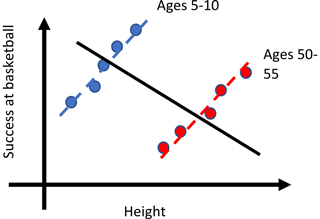Figure A1Demonstration of Simpson's paradox (Simpson1951) with the often-quoted relationship between “success at basketball” (y axis) and “height” (x axis). If data are separated by age, then the expected positive relationship emerges. If data are first aggregated, i.e., age is not taken into account, then a negative relationship emerges. The analogy to the current work is that aggregating blue points and red points separately and then fitting (as in the L3 methodology) may explain negative So values.

Appendix B

We address the flip in the relative slopes of low and high-fc points for L2 and L3 in Fig. 10. Based on Fig. 7 and Eq. (13), our intuition is that it is likely a function of the change in averaging–smoothing in Ac vs. averaging–smoothing in . To test this, we repeat Fig. 7 but now also look at smoothing in  and Nd.

What is apparent is that for L3, there is much more smoothing in Ac than in  at low fc (the L3 points lie closer to the 1:1 line in Fig. B1b vs. Fig. 7b for low fc). For L2, more low-fc points tend to lie below the 1:1 line in Fig. B1a vs. Fig. 7a, but because of this migration of points from above to below the line, it is more difficult to interpret. For L2N the smoothing in  and Ac looks similar, in line with our intuition (cf. Fig. 10, where one sees less of a bias in the relationship for low- and high-fc points).

To dig deeper, we look more closely at the σ ratios (Eq. 13) for (i) Ac, Nd (σ ratio Ac, Nd) and (ii) , Nd (σ ratio (, Nd) for the low-fc points. We then fit a linear relation between points for $\mathrm{0.3}<{f}_{\mathrm{c}}<\mathrm{0.43}$ (Fig. B2) and fc>0.85 (not shown) and obtain the following: for $\mathrm{0.3}<{f}_{\mathrm{c}}<\mathrm{0.43}$, L2: $y=\mathrm{0.06}+\mathrm{0.78}x$, L3: $y=-\mathrm{0.25}+\mathrm{1.03}x$, and L2N: $y=-\mathrm{0.22}+\mathrm{1.02}x$, with x the σ ratio for  and Nd and y the σ ratio for Ac and Nd. For fc>0.85, L2: $y=\mathrm{0.1}+\mathrm{0.90}x$, L3: $y=\mathrm{0.04}+\mathrm{0.94}x$, and L2N: $y=\mathrm{0.23}+\mathrm{0.81}x$.

The high-fc slopes for L2 and L3 are similar (0.90 vs. 0.94, respectively), but there is a more significant difference in the low-fc slopes (0.78 vs. 1.03 for L2 and L3, respectively) and in the direction consistent with the differences between L2 and L3 (Fig. 10a and b). Note the steepening of the low-fc points in L3 and the negative intercept for L3 at low fc (Fig. B2), which is consistent with Fig. 10b. This points strongly to the differences in degree of aggregation-related smoothing between Ac and  in these low-fc scenes being responsible for the flip in the relative sign of So vs.  adjustment slopes between Fig. 10a (L2) and Fig. 10b (L3).

We note that these differences in smoothing derive from the derivations of Ac (Eq. 4) and  (Eq. 3), with Ac a (nonlinear) function of τ only and  a function of the product of τ and re. Anticipating how the aggregation biases will play out is not intuitive and requires, in our experience, analyses of the kind shown here.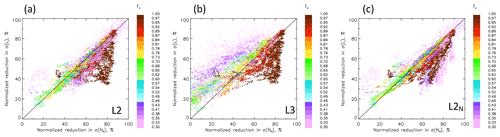Figure B1Effects of aggregation on normalized reduction in σ(ℒ) vs. normalized reduction in σ(Nd) to shed light on results in Fig. 10. Points are colored by fc. In (b), and at low fc, aggregation tends to less significantly reduce σ(ℒ) relative to σ(Ac) (Fig. 7b). (a) L2, (b) L3, and (c) L2N. See Appendix B for further discussion.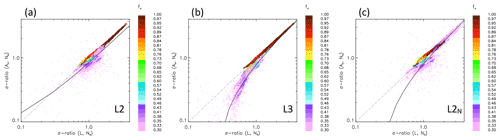Figure B2Plots of the σ ratio (Eq. 13) for Ac, Nd (σ ratio – Ac, Nd) vs. , Nd (σ ratio – , Nd). The solid lines are best-fit lines to the low-fc points. The dash-dotted line is the 1:1 line. Note that the log scale distorts the solid-line linear relationships. (a) L2, (b) L3, and (c) L2N. See Appendix B for further discussion.

Code and data availability

Model output is available on request.

Author contributions

The study was conceived by GF. GF and TG designed the study. GF performed the analysis. Simulations were done by TY. All the authors were engaged in reviewing results.

Competing interests

One of the authors is an editor of Atmospheric Chemistry and Physics. The peer-review process was guided by an independent editor, and the authors also have no other competing interests to declare.

Disclaimer

Publisher's note: Copernicus Publications remains neutral with regard to jurisdictional claims in published maps and institutional affiliations.

Acknowledgements

The authors are grateful for useful discussions with Jianhao Zhang and Yaosheng Chen.

Financial support

We thank the Department of Energy's Atmospheric System Research for supporting this work under Interagency Award 490 89243020SSC000055. Tom Goren was funded by German Research Foundation, Deutsche Forschungsgemeinschaft, DFG, project “CDNC4ACI”, GZ QU 311/27-1.

Review statement

This paper was edited by Timothy Garrett and reviewed by three anonymous referees.

References

Ackerman, A. S., Kirkpatrick, M. P., Stevens, D. E., and Toon, O. B.: The impact of humidity above stratiform clouds on indirect aerosol climate forcing, Nature, 432, 1014–1017, 2004.

Albrecht, B. A.: Aerosols, cloud microphysics, and fractional cloudiness, Science, 245, 1227–1230, 1989.

Bohren, C. F.: Multiple scattering of light and some of its observable consequences, Am. J. Phys., 55, 524–533, 1987.

Bony, S. and Dufresne, J.-L.: Marine boundary layer clouds at the heart of tropical cloud feedback uncertainties in climate models, Geophys. Res. Lett., 32, L20806, https://doi.org/10.1029/2005GL023851, 2005.

Bony, S., Stevens, B., Ament, F., Bigorre, S., Chazette, P., Crewell, S., Delanoë, J., Emanuel, K., Farrell, D., Flamant, C., Gross, S., Hirsch, L., Karstensen, J., Mayer, B., Nuijens, L., Ruppert Jr., J. H., Sandu, I., Siebesma, P., Speich, S., Szczap, F., Totems, J., Vogel, R., Wendisch, M., and Wirth, M.: EUREC4A: A Field Campaign to Elucidate the Couplings Between Clouds, Convection and Circulation, Surv. Geophys., 38, 1529–1568, https://doi.org/10.1007/s10712-017-9428-0, 2017.

Brenguier, J.-L., Pawlowska, H., Schüller, L., Preusker, R., Fischer, J., and Fouquart, Y.: Radiative Properties of Boundary Layer Clouds: Droplet Effective Radius versus Number Concentration, J. Atmos. Sci., 57, 803–821, https://doi.org/10.1175/1520-0469(2000)057<0803:RPOBLC>2.0.CO;2, 2000.

Cahalan, R. F., Ridgway, W., Wiscombe, W. J., Bell, T. L., and Snider, J. B.: The Albedo of Fractal Stratocumulus Clouds, J. Atmos. Sci., 51, 2434–2455, https://doi.org/10.1175/1520-0469(1994)051<2434:TAOFSC>2.0.CO;2, 1994.

Chen, Y.-C., Christensen, M., Stephens, G. L., and Seinfeld, J. H.: Satellite-based estimate of global aerosol–cloud radiative forcing by marine warm clouds, Nat. Geosci., 7, 643–646, https://doi.org/10.1038/ngeo2214, 2014.

Christensen, M. W. and Stephens, G. L.: Microphysical and macrophysical responses of marine stratocumulus polluted by underlying ships: Evidence of cloud deepening, J. Geophys. Res.-Atmos., 116, D03201, https://doi.org/10.1029/2010JD014638, 2011.

Feingold, G. and Levin, Z.: The lognormal fit to raindrop spectra from frontal convective clouds in Israel, J. Clim. Appl. Meteorol., 25, 1346–1363, 1986.

Feingold, G. and Siebert, H.: Cloud-aerosol interactions from the micro to the cloud scale, in: Clouds in the Perturbed Climate System, Strüngmann Forum Reports, edited by: Heintzenberg, J. and Charlson, R. J., MIT Press, ISBN 13: 9780262012874, https://doi.org/10.7551/mitpress/9780262012874.003.0014, 2009.

Feingold, G., Walko, R. L., Stevens, B., and Cotton, W. R.: Simulations of marine stratocumulus using a new microphysical parameterization scheme, Atmos. Res., 47–48, 505–528, https://doi.org/10.1016/S0169-8095(98)00058-1, 1998.

Feingold, G., McComiskey, A., Yamaguchi, T., Johnson, J. S., Carslaw, K., and Schmidt, K. S.: New approaches to quantifying aerosol influence on the cloud radiative effect, P. Natl. Acad. Sci. USA, 13, 5812–5819, https://doi.org/10.1073/pnas.1514035112, 2016.

Glassmeier, F., Hoffmann, F., Johnson, J. S., Yamaguchi, T., Carslaw, K. S., and Feingold, G.: Aerosol-cloud-climate cooling overestimated by ship-track data, Science, 371, 485–489, https://doi.org/10.1126/science.abd3980, 2021.

Grandey, B. S. and Stier, P.: A critical look at spatial scale choices in satellite-based aerosol indirect effect studies, Atmos. Chem. Phys., 10, 11459–11470, https://doi.org/10.5194/acp-10-11459-2010, 2010.

Grosvenor, D. P., Sourdeval, O., Zuidema, P., Ackerman, A., Alexandrov, M. D., Bennartz, R., Boers, R., Cairns, B., Chiu, J. C., Christensen, M., Deneke, H., Diamond, M., Feingold, G., Fridlind, A., Hinerbein, A., Knist, C., Kollias, P., Marshak, A., McCoy, D., Merk, D., Painemal, D., Rausch, J., Rosenfeld, D., Russchenberg, H., Seifert, P., Sinclair, K., Stier, P., van Diedenhoven, B., Wendisch, M., Werner, F., Wood, R., Zhang, Z., and Quaas, J.: Remote Sensing of Droplet Number Concentration in Warm Clouds: A Review of the Current State of Knowledge and Perspectives, Rev. Geophys., 56, 409–453, https://doi.org/10.1029/2017RG000593, 2018.

Gryspeerdt, E., Goren, T., Sourdeval, O., Quaas, J., Mülmenstädt, J., Dipu, S., Unglaub, C., Gettelman, A., and Christensen, M.: Constraining the aerosol influence on cloud liquid water path, Atmos. Chem. Phys., 19, 5331–5347, https://doi.org/10.5194/acp-19-5331-2019, 2019.

Hoffmann, F., Yamaguchi, T., and Feingold, G.: Inhomogeneous Mixing in Lagrangian Cloud Models: Effects on the Production of Precipitation Embryos, J. Atmos. Sci., 76, 113–133, https://doi.org/10.1175/JAS-D-18-0087.1, 2019.

Khairoutdinov, M. F. and Randall, D. A.: Cloud resolving modeling of the ARM summer 1997 IOP: Model formulation, results, uncertainties, and sensitivities, J. Atmos. Sci., 60, 607–625, 2003.

Lebsock, M., Morrison, H., and Gettelman, A.: Microphysical implications of cloud-precipitation covariance derived from satellite remote sensing, J. Geophys. Res.-Atmos., 118, 6521–6533, https://doi.org/10.1002/jgrd.50347, 2013.

Lebsock, M. D., Stephens, G. L., and Kummerow, C.: The retrieval of warm rain from CloudSat, J. Geophys. Res., 116, D20209, https://doi.org/10.1029/2011JD016076, 2008.

McComiskey, A. and Feingold, G.: The Scale Problem in Quantifying Aerosol Indirect Effects, Atmos. Chem. Phys., 12, 1031–1049, https://doi.org/10.5194/acp-12-1031-2012, 2012.

Norris, J. R. and Klein, S. A.: Low Cloud Type over the Ocean from Surface Observations. Part III: Relationship to Vertical Motion and the Regional Surface Synoptic Environment, J. Climate, 13, 245–256, https://doi.org/10.1175/1520-0442(2000)013<0245:LCTOTO>2.0.CO;2, 2000.

Oreopoulos, L. and Davies, R.: Plane Parallel Albedo Biases from Satellite Observations. Part I: Dependence on Resolution and Other Factors, J. Climate, 11, 919–932, https://doi.org/10.1175/1520-0442(1998)011<0919:PPABFS>2.0.CO;2, 1998.

Platnick, S. and Twomey, S.: Determining the susceptibility of cloud albedo to changes in droplet concentration with the advanced very high resolution radiometer, J. Appl. Meteorol., 33, 334–347, https://doi.org/10.1175/1520-0450(1994)033<0334:DTSOCA>2.0.CO;2, 1994.

Salomonson, V. V., Barnes, W. L., Maymon, P. W., Montgomery, H. E., and Ostrow, W.: Shallow Clouds, Water Vapor, Circulation, and Climate Sensitivity Shallow Clouds, Water Vapor, Circulation, and Climate Sensitivity, IEEE T. Geosci. Remote, 27, 145–153, https://doi.org/10.1109/36.20292, 1998.

Sharon, T. M., Albrecht, B. A., Jonsson, H. H., Minnis, P., Khaiyer, M. M., van Reken, T. M., Seinfeld, J., and Flagan, R.: Aerosol and Cloud Microphysical Characteristics of Rifts and Gradients in Maritime Stratocumulus Clouds, J. Atmos. Sci., 63, 983–997, https://doi.org/10.1175/JAS3667.1, 2006.

Simpson, E. H.: The Interpretation of Interaction in Contingency Tables, J. Roy. Stat. Soc. B, 13, 238–241, 1951.

Stevens, B., Vali, G., Comstock, K., Wood, R., van Zanten, M. C., Austin, P. H., Bretherton, C. S., and Lenschow, D. H.: Pockets of open cells and drizzle in marine stratocumulus, B. Am. Meteorol. Soc., 86, 51–58, https://doi.org/10.1175/BAMS-86-1-51, 2005.

Wang, H. and Feingold, G.: Modeling mesoscale cellular structures and drizzle in marine stratocumulus. Part I: Impact of drizzle on the formation and evolution of open cells, J. Atmos. Sci., 66, 3237–3256, https://doi.org/10.1175/2009JAS3022.1, 2009.

Wang, S., Wang, Q., and Feingold, G.: Turbulence, Condensation, and Liquid Water Transport in Numerically Simulated Nonprecipitating Stratocumulus Clouds, J. Atmos. Sci., 60, 262–278, https://doi.org/10.1175/1520-0469(2003)060<0262:TCALWT>2.0.CO;2, 2003.

Xue, H., Feingold, G., and Stevens, B.: Aerosol Effects on Clouds, Precipitation, and the Organization of Shallow Cumulus Convection, J. Atmos. Sci., 65, 392–406, https://doi.org/10.1175/2007JAS2428.1, 2008.

Zhang, Z., Song, H., Ma, P.-L., Larson, V. E., Wang, M., Dong, X., and Wang, J.: Subgrid variations of the cloud water and droplet number concentration over the tropical ocean: satellite observations and implications for warm rain simulations in climate models, Atmos. Chem. Phys., 19, 1077–1096, https://doi.org/10.5194/acp-19-1077-2019, 2019.